# Converting Between Fractions, Decimals, and Percents Lesson Plan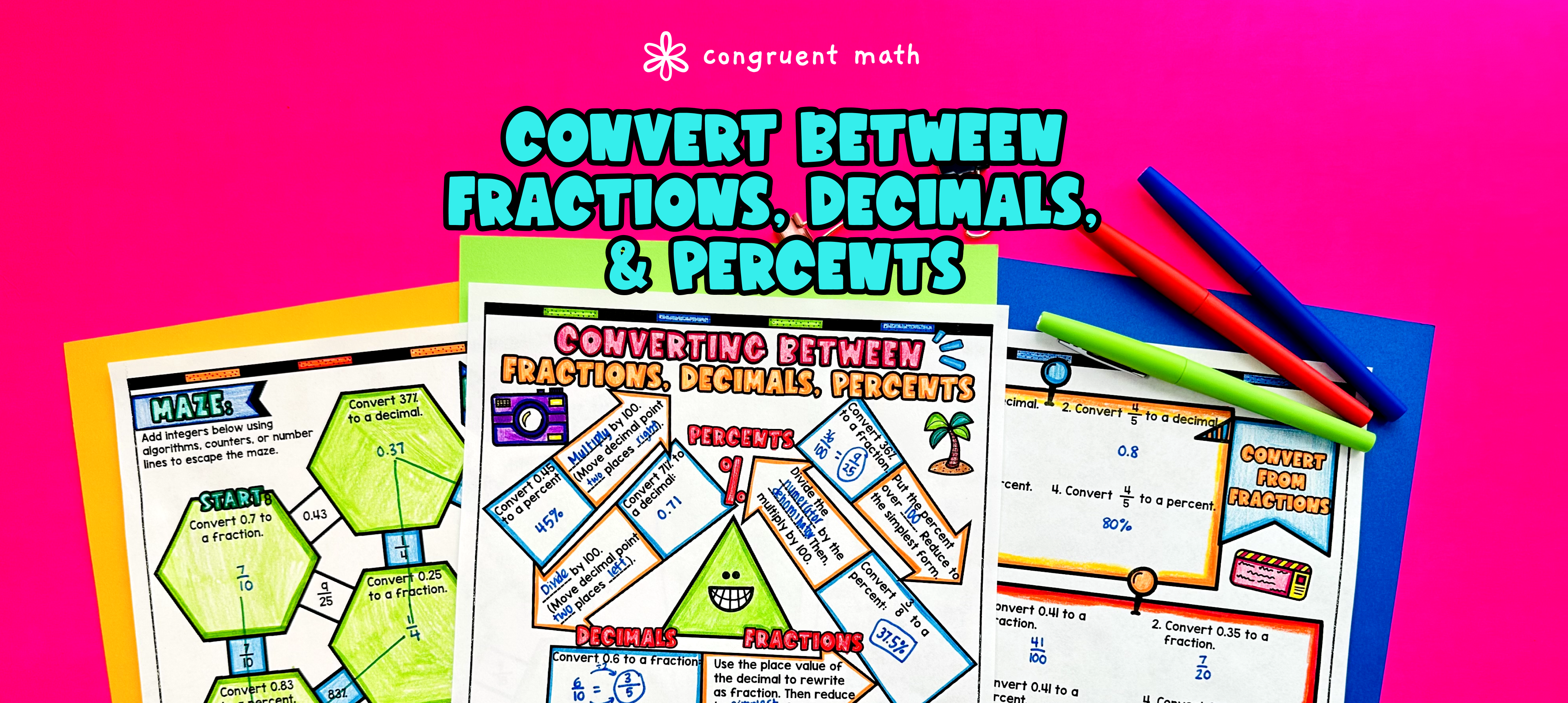Pin This

## Overview

Ever wondered how to teach converting between fractions, decimals, and percents in an engaging way to your seventh-grade students?

In this lesson plan, students will learn about converting between different forms rational numbers and their real-life applications. Through artistic, interactive guided notes, check for understanding, a doodle & color by number activity, and a maze worksheet, students will gain a comprehensive understanding of converting between fractions, decimals, and percentages.

The lesson culminates with a real-life example that explores how rational number conversions can be used in practical situations. Students will read and write about real-life uses of rational number conversions, allowing them to apply their newly acquired knowledge to a meaningful context.

## Get the Lesson Materials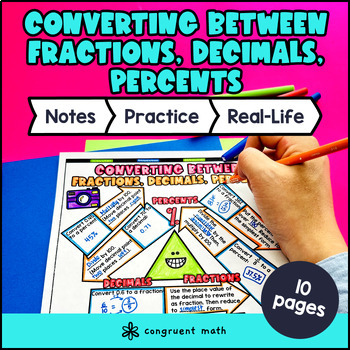\$4.25

## Learning Objectives

After this lesson, students will be able to:

• Convert fractions to decimals and vice versa

• Convert fractions to percentages and vice versa

• Convert decimals to percentages and vice versa

• Use long division and other methods to convert between rational numbers (decimals, fractions, and percents)

• Apply the concept of converting between rational numbers to real-life situations

## Prerequisites

Before this lesson, students should be familiar with:

• Basic operations of addition, subtraction, multiplication, and division of whole numbers

## Key Vocabulary

• Fractions

• Decimals

• Percents

• Rational Numbers

• Terminating Decimals

## Procedure

### Introduction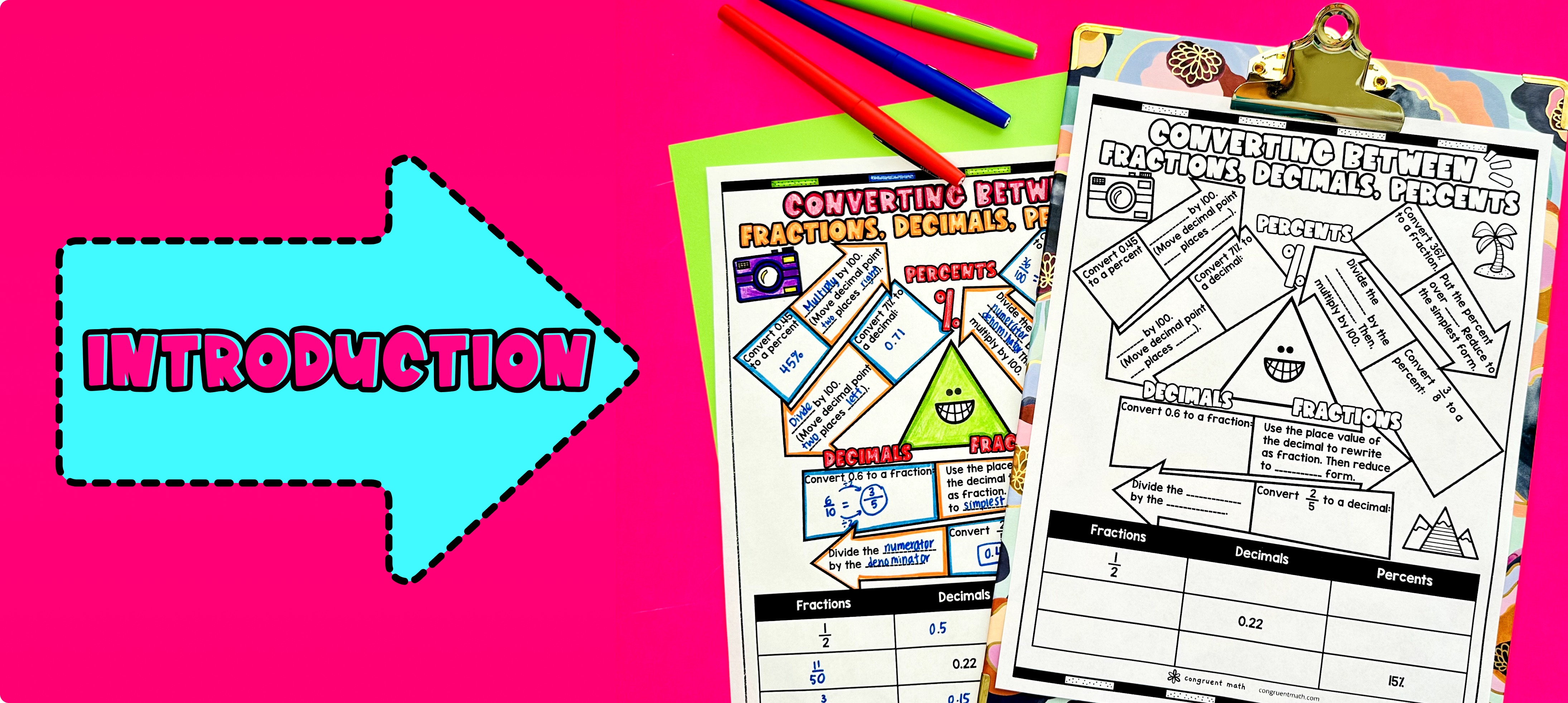Pin This

As a hook, ask students why it's important to be able to convert between fractions, decimals, and percents in real-life situations. Refer to the last page of the guided notes as well as the FAQs below for ideas.

Use the guided notes to introduce how to convert between rational numbers - decimals, fractions, and percents. Walk through the key points on the triangle conversion chart, including the steps and examples for converting between these different forms of numbers.

Based on student responses, reteach concepts that students need extra help with. If your class has a wide range of proficiency levels, you can pull out students for reteaching, and have more advanced students begin work on the practice exercises.

### Practice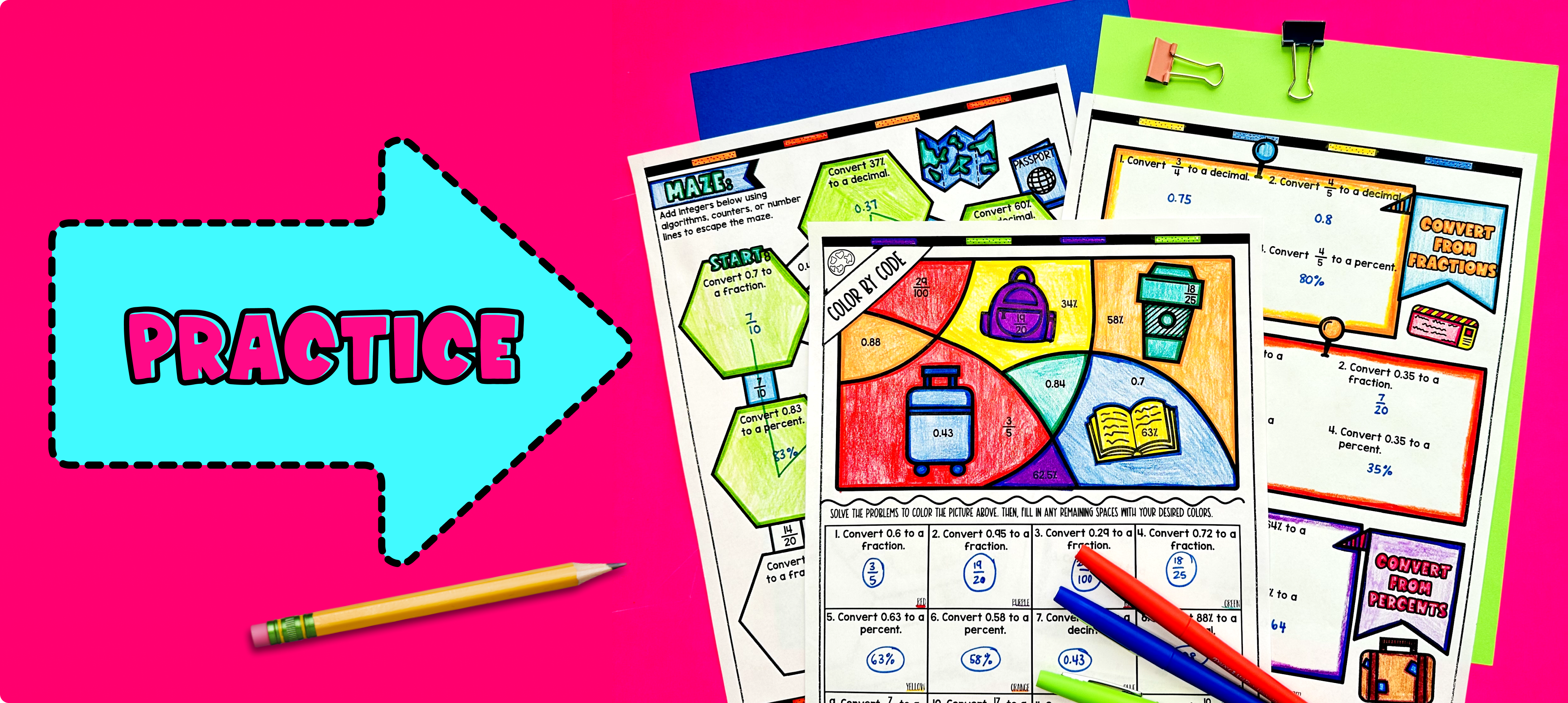Pin This

Have students practice converting between fractions, decimals, and percents using the practice worksheets provided in the resource (pg. 2). Walk around the classroom to answer any questions students may have and provide guidance as needed.

Fast finishers can dive into the maze or color by number activity included in the resource for extra practice. You can also assign it as homework for the remainder of the class.

### Real-Life Application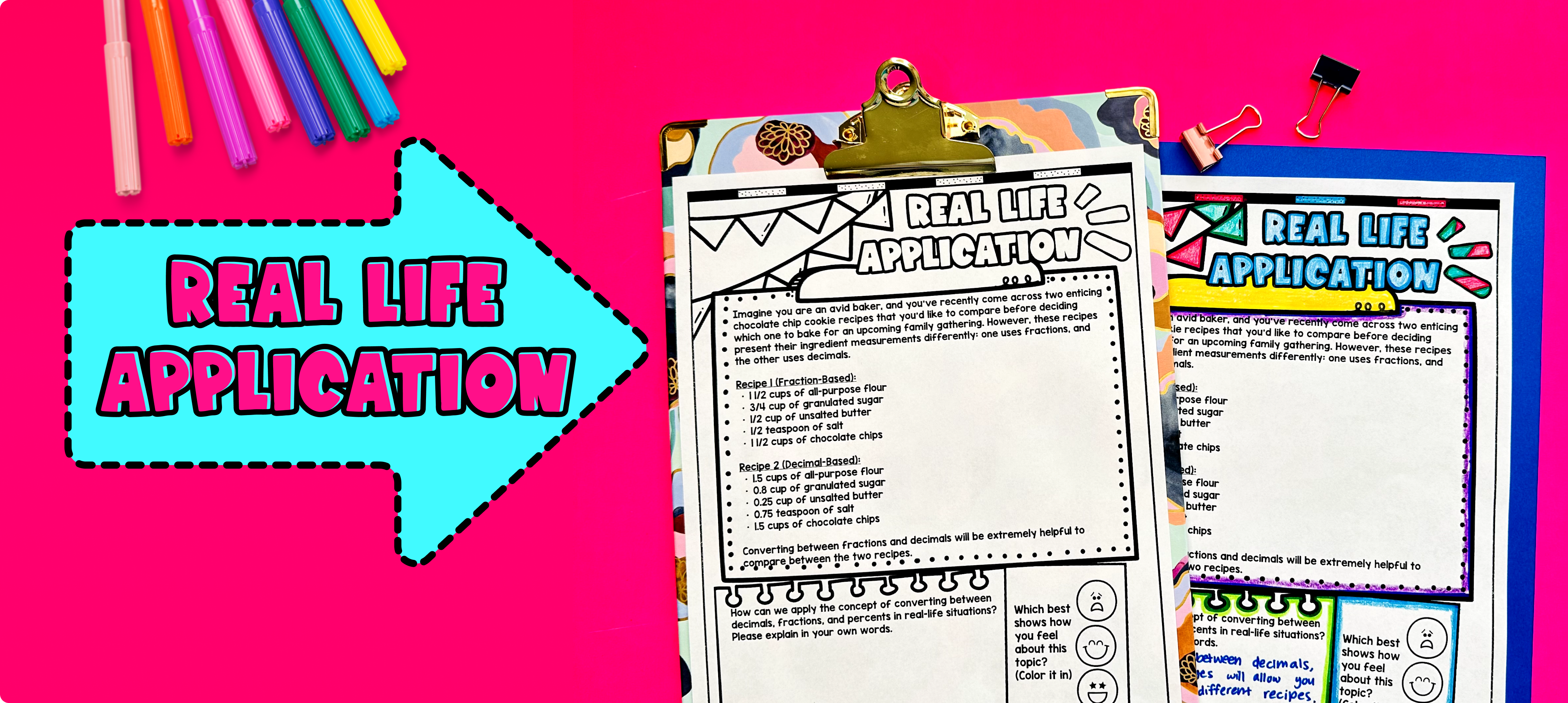Pin This

Bring the class back together, and introduce the concept of real-life uses of rational number conversions using the last page of the guided notes (pg. 5). Explain to students that converting between fractions, decimals, and percents is not just a math skill, but it has practical applications in our everyday lives.

One example is when we are cooking or baking and need to compare recipes. Sometimes recipes are written using fractions, while other times they are written using decimals. Being able to convert between these forms allows us to accurately measure ingredients and follow the recipe correctly.

Encourage students to think of other scenarios where converting between fractions, decimals, and percents is useful in their everyday lives.